Skip to main content
$$\require{cancel}$$

# 18: Electric Charge and Electric Field (Exercises)

## Conceptual Questions

#### 18.1: Static Electricity and Charge: Conservation of Charge

1. There are very large numbers of charged particles in most objects. Why, then, don’t most objects exhibit static electricity?

2. Why do most objects tend to contain nearly equal numbers of positive and negative charges?

#### 18.2: Conductors and Insulators

3. An eccentric inventor attempts to levitate by first placing a large negative charge on himself and then putting a large positive charge on the ceiling of his workshop. Instead, while attempting to place a large negative charge on himself, his clothes fly off. Explain.

4. If you have charged an electroscope by contact with a positively charged object, describe how you could use it to determine the charge of other objects. Specifically, what would the leaves of the electroscope do if other charged objects were brought near its knob?

5. When a glass rod is rubbed with silk, it becomes positive and the silk becomes negative—yet both attract dust. Does the dust have a third type of charge that is attracted to both positive and negative? Explain.

6. Why does a car always attract dust right after it is polished? (Note that car wax and car tires are insulators.)

7. Describe how a positively charged object can be used to give another object a negative charge. What is the name of this process?

8. What is grounding? What effect does it have on a charged conductor? On a charged insulator?

#### 18.3: Coulomb's Law

9. Figure shows the charge distribution in a water molecule, which is called a polar molecule because it has an inherent separation of charge. Given water’s polar character, explain what effect humidity has on removing excess charge from objects.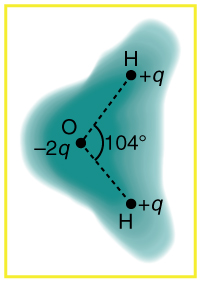Schematic representation of the outer electron cloud of a neutral water molecule. The electrons spend more time near the oxygen than the hydrogens, giving a permanent charge separation as shown. Water is thus a polar molecule. It is more easily affected by electrostatic forces than molecules with uniform charge distributions.

10. Using Figure, explain, in terms of Coulomb’s law, why a polar molecule (such as in Figure) is attracted by both positive and negative charges.

11. Given the polar character of water molecules, explain how ions in the air form nucleation centers for rain droplets.

#### 18.4: Electric Field: Concept of a Field Revisited

12. Why must the test charge $$q$$ in the definition of the electric field be vanishing small?

13. Are the direction and magnitude of the Coulomb force unique at a given point in space? What about the electric field?

#### 18.5: Electric Field Lines: Multiple Charges

14. Compare and contrast the Coulomb force field and the electric field. To do this, make a list of five properties for the Coulomb force field analogous to the five properties listed for electric field lines. Compare each item in your list of Coulomb force field properties with those of the electric field—are they the same or different? (For example, electric field lines cannot cross. Is the same true for Coulomb field lines?)

15. Figure shows an electric field extending over three regions, labeled I, II, and III. Answer the following questions.

(a) Are there any isolated charges? If so, in what region and what are their signs?

(b) Where is the field strongest?

(c) Where is it weakest?

(d) Where is the field the most uniform?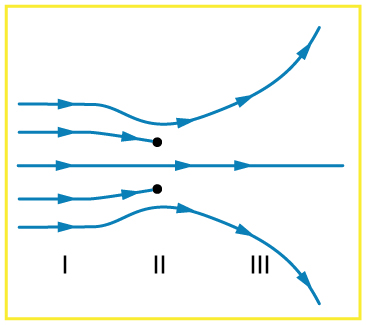#### 18.6: Electric Forces in Biology

16. A cell membrane is a thin layer enveloping a cell. The thickness of the membrane is much less than the size of the cell. In a static situation the membrane has a charge distribution of $$-2.5\times 10^{-6} C/m^{2}$$ on its inner surface and $$+2.5\times 10^{-6}C/m^{2}$$ on its outer surface. Draw a diagram of the cell and the surrounding cell membrane. Include on this diagram the charge distribution and the corresponding electric field. Is there any electric field inside the cell? Is there any electric field outside the cell?

#### 18.7: Conductors and Electric Fields in Static Equilibrium

17. Is the object in Figure 18.8.9 a conductor or an insulator? Justify your answer.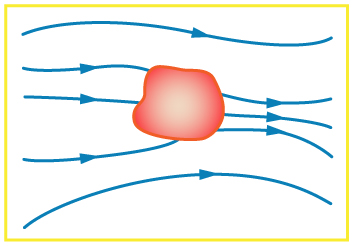18. If the electric field lines in the figure above were perpendicular to the object, would it necessarily be a conductor? Explain.

19. The discussion of the electric field between two parallel conducting plates, in this module states that edge effects are less important if the plates are close together. What does close mean? That is, is the actual plate separation crucial, or is the ratio of plate separation to plate area crucial?

20. Would the self-created electric field at the end of a pointed conductor, such as a lightning rod, remove positive or negative charge from the conductor? Would the same sign charge be removed from a neutral pointed conductor by the application of a similar externally created electric field? (The answers to both questions have implications for charge transfer utilizing points.)

21. Why is a golfer with a metal club over her shoulder vulnerable to lightning in an open fairway? Would she be any safer under a tree?

22. Can the belt of a Van de Graaff accelerator be a conductor? Explain.

23. Are you relatively safe from lightning inside an automobile? Give two reasons.

24. Discuss pros and cons of a lightning rod being grounded versus simply being attached to a building.

25. Using the symmetry of the arrangement, show that the net Coulomb force on the charge $$q$$ at the center of the square below (Figure) is zero if the charges on the four corners are exactly equal.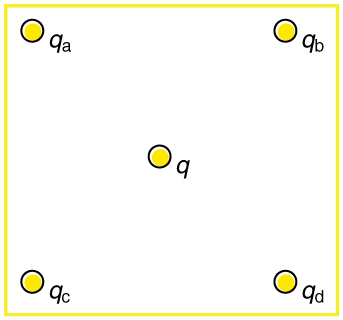Four point charges $$q_{a}$$, $$q_{b}$$, $$q_{c}$$, and $$q_{d}$$ lie on the corners of a square and $$q$$ is located at its center.

(a) Using the symmetry of the arrangement, show that the electric field at the center of the square in Figure zero if the charges on the four corners are exactly equal.

(b) Show that this is also true for any combination of charges in which $$q_{a}=q_{d}$$ and $$q_{b}=q_{c}$$

26. (a) What is the direction of the total Coulomb force on $$q$$ in Figure if $$q$$ is negative, $$q_{a}= q_{c}$$ and both are negative, and $$q_{b}=q_{c}$$ and both are positive? (b) What is the direction of the electric field at the center of the square in this situation?

27. Considering Figure, suppose that $$q_{a}=q_{d}$$ and $$q_{b}=q_{c}$$. First show that $$q$$ is in static equilibrium. (You may neglect the gravitational force.) Then discuss whether the equilibrium is stable or unstable, noting that this may depend on the signs of the charges and the direction of displacement of $$q$$ from the center of the square.

28. If $$q_{a}=0$$ in Figure, under what conditions will there be no net Coulomb force on $$q$$?

29. In regions of low humidity, one develops a special “grip” when opening car doors, or touching metal door knobs. This involves placing as much of the hand on the device as possible, not just the ends of one’s fingers. Discuss the induced charge and explain why this is done.

30. Tollbooth stations on roadways and bridges usually have a piece of wire stuck in the pavement before them that will touch a car as it approaches. Why is this done?

31. Suppose a woman carries an excess charge. To maintain her charged status can she be standing on ground wearing just any pair of shoes? How would you discharge her? What are the consequences if she simply walks away?

## Problems & Exercises

#### 18.1: Static Electricity and Charge: Conservation of Charge

32. Common static electricity involves charges ranging from nanocoulombs to microcoulombs.

(a) How many electrons are needed to form a charge of $$–2.00nC$$

(b) How many electrons must be removed from a neutral object to leave a net charge of $$0.500\mu C$$ ?

Solution
a. $$1.25\times 10^{10}$$
b. $$3.13\times 10^{12}$$

33. If $$1.80\times 10^{20}$$ electrons move through a pocket calculator during a full day’s operation, how many coulombs of charge moved through it?

34. To start a car engine, the car battery moves $$3.75\times 10^{21}$$ electrons through the starter motor. How many coulombs of charge were moved?

Solution
-600C

35. A certain lightning bolt moves 40.0 C of charge. How many fundamental units of charge $$|q_{e}|$$ is this?

#### 18.2: Conductors and Insulators

36. Suppose a speck of dust in an electrostatic precipitator has $$1.0000\times 10^{12}$$ protons in it and has a net charge of –5.00 nC (a very large charge for a small speck). How many electrons does it have?

Solution
$$1.03\times 10^{12}$$

37. An amoeba has $$1.00\times 10^{16}$$ protons and a net charge of 0.300 pC. (a) How many fewer electrons are there than protons? (b) If you paired them up, what fraction of the protons would have no electrons?

38. A 50.0 g ball of copper has a net charge of $$2.00\mu C$$. What fraction of the copper’s electrons has been removed? (Each copper atom has 29 protons, and copper has an atomic mass of 63.5.)

Solution
$$9.09\times 10^{-13}$$

39. What net charge would you place on a 100 g piece of sulfur if you put an extra electron on 1 in $$10^{12}$$ of its atoms? (Sulfur has an atomic mass of 32.1.)

40. How many coulombs of positive charge are there in 4.00 kg of plutonium, given its atomic mass is 244 and that each plutonium atom has 94 protons?

Solution
$$1.48\times 10^{8}C$$

#### 18.3: Coulomb's Law

41. What is the repulsive force between two pith balls that are 8.00 cm apart and have equal charges of – 30.0 nC?

42. (a) How strong is the attractive force between a glass rod with a $$0.700\mu C$$ charge and a silk cloth with a $$-0.600\mu C$$ charge, which are 12.0 cm apart, using the approximation that they act like point charges?

(b) Discuss how the answer to this problem might be affected if the charges are distributed over some area and do not act like point charges.

Solution
(a) 0.263 N
(b) If the charges are distributed over some area, there will be a concentration of charge along the side closest to the oppositely charged object. This effect will increase the net force.

43. Two point charges exert a 5.00 N force on each other. What will the force become if the distance between them is increased by a factor of three?

44. Two point charges are brought closer together, increasing the force between them by a factor of 25. By what factor was their separation decreased?

Solution
The separation decreased by a factor of 5.

45. How far apart must two point charges of 75.0 nC (typical of static electricity) be to have a force of 1.00 N between them?

46. If two equal charges each of 1 C each are separated in air by a distance of 1 km, what is the magnitude of the force acting between them? You will see that even at a distance as large as 1 km, the repulsive force is substantial because 1 C is a very significant amount of charge.

47. A test charge of $$+2\mu C$$ is placed halfway between a charge of $$+6\mu C$$ and another of $$+4\mu C$$ separated by 10 cm.

(a) What is the magnitude of the force on the test charge?

(b) What is the direction of this force (away from or toward the $$+6\mu C$$ charge)?

48. Bare free charges do not remain stationary when close together. To illustrate this, calculate the acceleration of two isolated protons separated by 2.00 nm (a typical distance between gas atoms). Explicitly show how you follow the steps in the Problem-Solving Strategy for electrostatics.

Solution
$F=k\dfrac{|q_{1}q_{2}|}{r^{2}}=ma\Rightarrow a=\dfrac{kq^{2}}{mr^{2}}$
$=\dfrac{(9.00\times 10^{9}N\cdot m^{2}/C^{2})(1.60\times 10^{-19}m)^{2}}{(1.67\times 10^{-27}kg)(2.00\times 10^{-9}m)^{2}}$
$=3.45\times 10^{16}m/s^{2}$

49. (a) By what factor must you change the distance between two point charges to change the force between them by a factor of 10?

(b) Explain how the distance can either increase or decrease by this factor and still cause a factor of 10 change in the force.

Solution
(a) 3.2
(b) If the distance increases by 3.2, then the force will decrease by a factor of 10 ; if the distance decreases by 3.2, then the force will increase by a factor of 10. Either way, the force changes by a factor of 10.

50. Suppose you have a total charge $$q_{tot}$$ that you can split in any manner. Once split, the separation distance is fixed. How do you split the charge to achieve the greatest force?

51. (a) Common transparent tape becomes charged when pulled from a dispenser. If one piece is placed above another, the repulsive force can be great enough to support the top piece’s weight. Assuming equal point charges (only an approximation), calculate the magnitude of the charge if electrostatic force is great enough to support the weight of a 10.0 mg piece of tape held 1.00 cm above another.

(b) Discuss whether the magnitude of this charge is consistent with what is typical of static electricity.

Solution
(a) $$1.04\times 10^{-9}C$$
(b) This charge is approximately 1 nC, which is consistent with the magnitude of charge typical for static electricity

52. (a) Find the ratio of the electrostatic to gravitational force between two electrons.

(b) What is this ratio for two protons?

(c) Why is the ratio different for electrons and protons?

53. At what distance is the electrostatic force between two protons equal to the weight of one proton?

54. A certain five cent coin contains 5.00 g of nickel. What fraction of the nickel atoms’ electrons, removed and placed 1.00 m above it, would support the weight of this coin? The atomic mass of nickel is 58.7, and each nickel atom contains 28 electrons and 28 protons.

Solution
$$1.02\times 10^{-11}$$

55. (a) Two point charges totaling $$8.00 \mu C$$ exert a repulsive force of 0.150 N on one another when separated by 0.500 m. What is the charge on each?

(b) What is the charge on each if the force is attractive?

56. Point charges of $$5.00 \mu C$$ and $$-3.00 \mu C$$ are placed 0.250 m apart.

(a) Where can a third charge be placed so that the net force on it is zero?

(b) What if both charges are positive?

Solution
(a). 0.859 m beyond negative charge on line connecting two charges
(b). 0.109 m from lesser charge on line connecting two charges

57. Two point charges $$q_{1}$$ and $$q_{2}$$ are $$3.00m$$ apart, and their total charge is $$20\mu C$$.

(a) If the force of repulsion between them is 0.075N, what are magnitudes of the two charges?

(b) If one charge attracts the other with a force of 0.525N, what are the magnitudes of the two charges? Note that you may need to solve a quadratic equation to reach your answer.

#### 18.4: Electric Field: Concept of a Field Revisited

58. What is the magnitude and direction of an electric field that exerts a $$2.00\times 10^{-5} N$$ upward force on a $$-1.75 \mu C$$ charge?

59. What is the magnitude and direction of the force exerted on a $$3.50\mu C$$ charge by a 250 N/C electric field that points due east?

Solution
$$8.75\times 10^{-4}$$

60. Calculate the magnitude of the electric field 2.00 m from a point charge of 5.00 mC (such as found on the terminal of a Van de Graaff).

61. (a) What magnitude point charge creates a 10,000 N/C electric field at a distance of 0.250 m?

(b) How large is the field at 10.0 m?

Solution
(a) $$6.94\times 10^{-8}C$$
(b) $$6.25N/C$$

62. Calculate the initial (from rest) acceleration of a proton in a $$5.00\times 10^{6} N/C$$ electric field (such as created by a research Van de Graaff). Explicitly show how you follow the steps in the Problem-Solving Strategy for electrostatics.

63. (a) Find the direction and magnitude of an electric field that exerts a $$4.80\times 10^{-17}N$$westward force on an electron.

(b) What magnitude and direction force does this field exert on a proton?

Solution
(a) 300N/C (east)
(b) $$4.80\times 10^{-17}N$$ (east)

#### 18.5: Electric Field Lines: Multiple Charges

64. (a) Sketch the electric field lines near a point charge $$+q$$.

(b) Do the same for a point charge $$-3.00q$$.

65. Sketch the electric field lines a long distance from the charge distributions shown in Figure (a) and (b)

66. Figure shows the electric field lines near two charges $$q_1$$ and $$q_2$$. What is the ratio of their magnitudes?

(b) Sketch the electric field lines a long distance from the charges shown in the figure.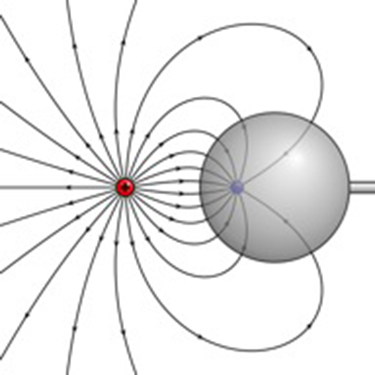The electric field near two charges.

67. Sketch the electric field lines in the vicinity of two opposite charges, where the negative charge is three times greater in magnitude than the positive. (See Figure 18.6.8 for a similar situation).

#### 18.7: Conductors and Electric Fields in Static Equilibrium

68. Sketch the electric field lines in the vicinity of the conductor in Figure given the field was originally uniform and parallel to the object’s long axis. Is the resulting field small near the long side of the object?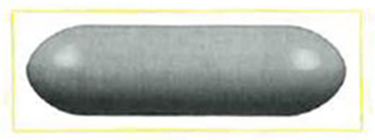69. Sketch the electric field lines in the vicinity of the conductor in Figure 18.8.12 given the field was originally uniform and parallel to the object’s long axis. Is the resulting field small near the long side of the object?70. Sketch the electric field between the two conducting plates shown in Figure, given the top plate is positive and an equal amount of negative charge is on the bottom plate. Be certain to indicate the distribution of charge on the plates.71. Sketch the electric field lines in the vicinity of the charged insulator in Figure noting its nonuniform charge distribution.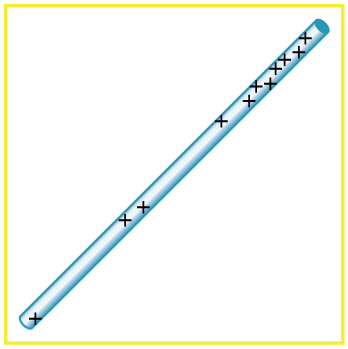A charged insulating rod such as might be used in a classroom demonstration.

72. What is the force on the charge located at $$x=8.00cm$$ in Figure (a) given that $$q=1.00 \mu C$$?(a) Point charges located at 3.00, 8.00, and 11.0 cm along the x-axis. (b) Point charges located at 1.00, 5.00, 8.00, and 14.0 cm along the x-axis.

73. (a) Find the total electric field at $$x=1.00cm$$ in Figure

(b) given that $$q=5.00nC$$. (b) Find the total electric field at $$x=1.00cm$$ in Figure 18.8.15(b).

(c) If the charges are allowed to move and eventually be brought to rest by friction, what will the final charge configuration be? (That is, will there be a single charge, double charge, etc., and what will its value(s) be?)

Solution
(a) $$E_{x=1.00cm}=-\infty$$
(b) $$2.12\times 10^{5} N/C$$
(c) one charge of $$+q$$

74. (a) Find the electric field at $$x=5.00com$$ in Figure 18.8.15(a), given that $$q=1.00\mu C$$.

(b) At what position between 3.00 and 8.00 cm is the total electric field the same as that for $$-2q$$ alone?

(c) Can the electric field be zero anywhere between 0.00 and 8.00 cm?

(d) At very large positive or negative values of x, the electric field approaches zero in both (a) and (b). In which does it most rapidly approach zero and why?

(e) At what position to the right of 11.0 cm is the total electric field zero, other than at infinity? (Hint: A graphing calculator can yield considerable insight in this problem.)

75. (a) Find the total Coulomb force on a charge of 2.00 nC located at $$x=4.00cm$$ in Figure

(b), given that $$q=1.00\mu C$$. (b) Find the x-position at which the electric field is zero in Fgiure 18.8.15(b).

Solution
(a) 0.252 N to the left
(b) $$x=6.07cm$$

76. Using the symmetry of the arrangement, determine the direction of the force on $$q$$ in the figure below, given that $$q_{a}=q_{b}=+7.50 \mu C$$ and $$q_{c}=q_{d}=-7.50 \mu C$$.

(b) Calculate the magnitude of the force on the charge $$q$$, given that the square is 10.0 cm on a side and $$q=2.00 \mu C$$.77. (a) Using the symmetry of the arrangement, determine the direction of the electric field at the center of the square in Figure 18.8.16, given that $$q_{a}=q_{b}=-1.00 \mu C$$ and $$q_{c}=q_{d}=+1.00 \mu C$$.

(b) Calculate the magnitude of the electric field at the location of $$q$$, given that the square is 5.00 cm on a side.

Solution
(a) The electric field at the center of the square will be straight up, since $$q_{a}$$ and $$q_{b}$$ are positive and $$q_{c}$$ and $$q_{d}$$are negative and all have the same magnitude.
(b) $$2.04\times 10^{7} N/C$$ (upward)

78. Find the electric field at the location of $$q_{a}$$ in Figure 18.8.16 given that $$q_{b}=q_{c}=q_{d}=+2.00nC$$, $$q=-1.00nC$$, and the square is 20.0 cm on a side.

79. Find the total Coulomb force on the charge $$q$$ in Figure 18.8.16, given that $$q=1.00\mu C$$, $$q_{a}=2.00\mu C$$, $$q_{b}=-3.00 \mu C$$, $$q_{c}=-4.00\mu C$$, and $$q_{d}=+1.00 \mu C$$. The square is 50.0 cm on a side.

Solution
0.102 N, in the $$-y$$ direction

80. (a) Find the electric field at the location of $$q_{a}$$ in Figure, given that $$q_{b}=+10.00 \mu C$$ and $$q_{c}=-5.00\mu C$$.

(b) What is the force on $$q_{a}$$, given that $$q_{a}=+1.50nC$$?Point charges located at the corners of an equilateral triangle 25.0 cm on a side.

81. (a) Find the electric field at the center of the triangular configuration of charges in Figure 18.8.17, given that $$q_{a}=+2.50nC$$, $$q_{b}=-8.00nC$$, and $$q_{c}=+1.50nC$$.

(b) Is there any combination of charges, other than $$q_{a}=q_{b}=q_{c}$$, that will produce a zero strength electric field at the center of the triangular configuration?

Solution
(a) $$\overrightarrow{E}=4.36\times 10^{3} N/C, 35.0^{\circ}$$, below the horizontal.
(b) No

#### 18.8: Applications of Electrostatics

82. (a) What is the electric field 5.00 m from the center of the terminal of a Van de Graaff with a 3.00 mC charge, noting that the field is equivalent to that of a point charge at the center of the terminal?

(b) At this distance, what force does the field exert on a $$2.00 \mu C$$ charge on the Van de Graaff’s belt?

83. (a) What is the direction and magnitude of an electric field that supports the weight of a free electron near the surface of Earth?

(b) Discuss what the small value for this field implies regarding the relative strength of the gravitational and electrostatic forces.

Solution
(a) $$5.58\times 10^{-11} N/C$$
(b) the coulomb force is extraordinarily stronger than gravity

84. A simple and common technique for accelerating electrons is shown in Figure, where there is a uniform electric field between two plates. Electrons are released, usually from a hot filament, near the negative plate, and there is a small hole in the positive plate that allows the electrons to continue moving. (a) Calculate the acceleration of the electron if the field strength is $$2.50 \times 10^[4}N/C$$.

(b) Explain why the electron will not be pulled back to the positive plate once it moves through the hole.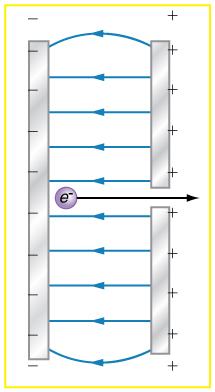Parallel conducting plates with opposite charges on them create a relatively uniform electric field used to accelerate electrons to the right. Those that go through the hole can be used to make a TV or computer screen glow or to produce X-rays.

85. Earth has a net charge that produces an electric field of approximately 150 N/C downward at its surface.

(a) What is the magnitude and sign of the excess charge, noting the electric field of a conducting sphere is equivalent to a point charge at its center?

(b) What acceleration will the field produce on a free electron near Earth’s surface?

(c) What mass object with a single extra electron will have its weight supported by this field?

Solution
(a) $$-6.76\times 10^{5}C$$
(b) $$2.63\times 10^{13} m/s^{2}$$ (upward)
(c) $$2.45\times 10^{-18}kg$$

86. Point charges of $$25.0 \mu C$$ and $$45.0 \mu C$$ are placed 0.500 m apart.

(a) At what point along the line between them is the electric field zero?

(b) What is the electric field halfway between them?

87. What can you say about two charges $$q_{1}$$ and $$q_{2}$$, if the electric field one-fourth of the way from $$q_{1}$$ to $$q_{2}$$ is zero?

Solution
The charge $$q_{2}$$ is 9 times greater than $$q_{1}$$.

88. Calculate the angular velocity $$\omega$$ of an electron orbiting a proton in the hydrogen atom, given the radius of the orbit is $$0.530\times 10^{-10}m$$. You may assume that the proton is stationary and the centripetal force is supplied by Coulomb attraction.

89. An electron has an initial velocity of $$5.00\times 10^{6}m/s$$ in a uniform $$2.00\times 10^{5} N/C$$ strength electric field. The field accelerates the electron in the direction opposite to its initial velocity.

(a) What is the direction of the electric field?

(b) How far does the electron travel before coming to rest?

(c) How long does it take the electron to come to rest? (d) What is the electron’s velocity when it returns to its starting point?

90. The practical limit to an electric field in air is about $$3.00\times 10^{6}N/C$$. Above this strength, sparking takes place because air begins to ionize and charges flow, reducing the field.

(a) Calculate the distance a free proton must travel in this field to reach$$3.00%$$ of the speed of light, starting from rest.

(b) Is this practical in air, or must it occur in a vacuum?

91. A 5.00 g charged insulating ball hangs on a 30.0 cm long string in a uniform horizontal electric field as shown in Figure. Given the charge on the ball is $$1.00 \mu C$$, find the strength of the field.A horizontal electric field causes the charged ball to hang at an angle of $$8.00^{\circ}$$.

92. Figure shows an electron passing between two charged metal plates that create an 100 N/C vertical electric field perpendicular to the electron’s original horizontal velocity. (These can be used to change the electron’s direction, such as in an oscilloscope.) The initial speed of the electron is $$3.00\times 10^{6}m/s$$, and the horizontal distance it travels in the uniform field is 4.00 cm.

(a) What is its vertical deflection?

(b) What is the vertical component of its final velocity?

(c) At what angle does it exit? Neglect any edge effects.93. The classic Millikan oil drop experiment was the first to obtain an accurate measurement of the charge on an electron. In it, oil drops were suspended against the gravitational force by a vertical electric field. (Figure 18.9.9) Given the oil drop to be $$1.00\mu m$$ in radius and have a density of $$920 kg/m^{3}$$: (a) Find the weight of the drop. (b) If the drop has a single excess electron, find the electric field strength needed to balance its weight.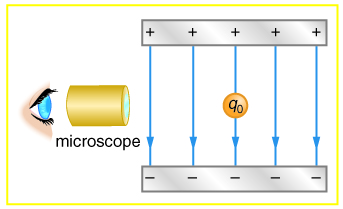In the Millikan oil drop experiment, small drops can be suspended in an electric field by the force exerted on a single excess electron. Classically, this experiment was used to determine the electron charge $$q_{e}$$ by measuring the electric field and mass of the drop.

94. (a) In Figure, four equal charges $$q$$ lie on the corners of a square. A fifth charge $$Q$$ is on a mass $$m$$ directly above the center of the square, at a height equal to the length $$d$$ of one side of the square. Determine the magnitude of $$q$$ in terms of $$Q$$,$$m$$, and $$d$$, if the Coulomb force is to equal the weight of $$m$$. (b) Is this equilibrium stable or unstable? Discuss.Four equal charges on the corners of a horizontal square support the weight of a fifth charge located directly above the center of the square.

95. (a) Calculate the electric field strength near a 10.0 cm diameter conducting sphere that has 1.00 C of excess charge on it.

(b) What is unreasonable about this result? (c) Which assumptions are responsible?

96. (a) Two 0.500 g raindrops in a thunderhead are 1.00 cm apart when they each acquire 1.00 mC charges. Find their acceleration.

(b) What is unreasonable about this result?

(c) Which premise or assumption is responsible?

97. A wrecking yard inventor wants to pick up cars by charging a 0.400 m diameter ball and inducing an equal and opposite charge on the car. If a car has a 1000 kg mass and the ball is to be able to lift it from a distance of 1.00 m:

(a) What minimum charge must be used?

(b) What is the electric field near the surface of the ball?

(c) Why are these results unreasonable?

(d) Which premise or assumption is responsible?

98. Consider two insulating balls with evenly distributed equal and opposite charges on their surfaces, held with a certain distance between the centers of the balls. Construct a problem in which you calculate the electric field (magnitude and direction) due to the balls at various points along a line running through the centers of the balls and extending to infinity on either side. Choose interesting points and comment on the meaning of the field at those points. For example, at what points might the field be just that due to one ball and where does the field become negligibly small? Among the things to be considered are the magnitudes of the charges and the distance between the centers of the balls. Your instructor may wish for you to consider the electric field off axis or for a more complex array of charges, such as those in a water molecule.

99. Consider identical spherical conducting space ships in deep space where gravitational fields from other bodies are negligible compared to the gravitational attraction between the ships. Construct a problem in which you place identical excess charges on the space ships to exactly counter their gravitational attraction. Calculate the amount of excess charge needed. Examine whether that charge depends on the distance between the centers of the ships, the masses of the ships, or any other factors. Discuss whether this would be an easy, difficult, or even impossible thing to do in practice.

### Contributors

• Paul Peter Urone (Professor Emeritus at California State University, Sacramento) and Roger Hinrichs (State University of New York, College at Oswego) with Contributing Authors: Kim Dirks (University of Auckland) and Manjula Sharma (University of Sydney). This work is licensed by OpenStax University Physics under a Creative Commons Attribution License (by 4.0).